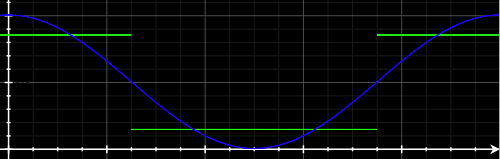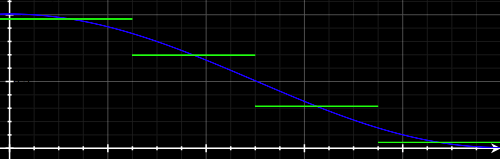# Discrete Cosine Transforms Part 1

I've often been intrigued by the lossy part of JPEG compression so I thought I'd explore Discrete Cosine Transforms and their use in JPEG as a short multi-part blog series.

In this part, lets just talk about what a "discrete cosine" function is and then in the next couple of posts look at how the concept can be combined with basic linear algebra to break up images into components in such a way that you can throw out some components with minimal effect on the perceived image. It's quite clever but the mathematics is fairly straightforward.

Let's start with a single cycle of a cosine function shifted up and scaled so its values range from 0-255 instead of from -1 to +1. To make it discrete, we'll divide it up into four and simply take the value of the scaled cosine function at the midpoint of each of our four sections:Here our four discrete values are 217, 39, 39, 217 .

Now let's do the same for one and a half cycles:which yields values of 176, 11, 245 and 80.

Now half a cycle:which yields 245, 176, 80 and 11 and zero cycles:which gives us 255, 255, 255, 255

We've basically calculated values for 0 thru 3 half cycles. If we wanted to split the cosine into N pieces instead of 4, we'd calculate values for 0 thru N-1 half cycles.

But, in summary, for N = 4:

• k = 3: 176, 11, 245, 80
• k = 2: 217, 39, 39, 217
• k = 1: 245, 176, 80, 11
• k = 0: 255, 255, 255, 255

The original post was in the category: mathematics but I'm still in the process of migrating categories over.